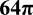# Diagonal of a Cube

Back to “Geometry” Page

The diagonal of a cube is nearly as simple as the Pythagorean theorem.

It is very simple:

(length)2 + (width)2 + (height)2 = (diagonal)2

In other words, you simply take the square root of the sum of the squares of the length, width, and height of the cube.

(length)2 + (width)2 + (height)2   = d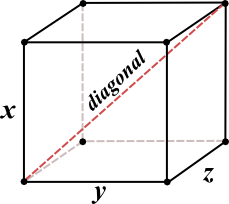A simple equation for the diagram above would be this:

x2 + y2 + z2   = d

Since the length, width, and height of a cube are equal, you can simply multiply one side squared by 3, like this:

3(x2) = d

So, if a side of the cube above is equal to 1, then the equation for the diagonal is this:

3(12) = d

3  = d

It is handy to remember that  3  is approximately 1.7 or so. Just keep that tucked away in your memory.

And, if a side of the cube above is equal to 2, then the equation for the diagonal is this:

3(22) = d

3(4)  = d

2 3  = d

And, if a side of the cube above is equal to 3, then the equation for the diagonal is this:

3(32) = d

3(9)  = d

3 3  = d

You can now see a pattern developing. The diagonal of a cube is equal to any side of the cube times  3 .

See whether you can solve the flashcards below.• ﻿What is the diagonal the cube above?;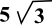• What is the area of one face of the cube? ; 52 or 25
• What is the surface area of the cube?; 6(25) or 150
• What is the volume of the cube? ; 53 or 125• ﻿What is the diagonal of the cube above?;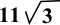• What is the area of one face of the cube? ; 112 or 121
• What is the surface area of the cube?; 6(121) or 726
• What is the volume of the cube? ; 113 or 1,331• ﻿What is the diagonal of the cube above?;• What is the area of one face of the cube? ; 272 or 729
• What is the surface area of the cube?; 6(729) or 4,374
• What is the volume of the cube? ; 273 or 19,683Assume that the sphere touches all six sides of the cube in the diagram above.

• ﻿What's the diameter of the sphere?; 6
• What is the area of one face of the cube? ; 62 or 36
• What is the surface area of the cube?; 6(36) or 216
• What is the volume of the cube? ; 63 or 216

WAIT! WAIT! Not done yet!
Recall the formula for volume of a sphere:

V = 4/3 π r3

And the formula for surface area of a sphere:

A = 4π r2

• ﻿What's the volume of the sphere?;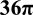• What's the surface area of the sphere?;Now, try one more diagram. Remember that standardized tests often give answer choices with fractions, roots and π symbols in the answer.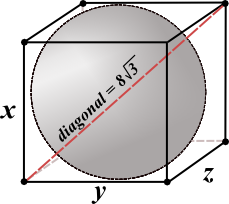Assume that the sphere touches all six sides of the cube in the diagram above.

• ﻿What's the diameter of the sphere?; 8
• What is the area of one face of the cube? ; 82 or 64
• What is the surface area of the cube?; 6(64) or 384
• What is the volume of the cube? ; 83 or 512
• What's the volume of the sphere?;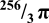• What's the surface area of the sphere?;